NEET  >  Test: Electric Field Intensity & Electric Field Calculations

# Test: Electric Field Intensity & Electric Field Calculations

Test Description

## 20 Questions MCQ Test Physics Class 12 | Test: Electric Field Intensity & Electric Field Calculations

Test: Electric Field Intensity & Electric Field Calculations for NEET 2023 is part of Physics Class 12 preparation. The Test: Electric Field Intensity & Electric Field Calculations questions and answers have been prepared according to the NEET exam syllabus.The Test: Electric Field Intensity & Electric Field Calculations MCQs are made for NEET 2023 Exam. Find important definitions, questions, notes, meanings, examples, exercises, MCQs and online tests for Test: Electric Field Intensity & Electric Field Calculations below.
Solutions of Test: Electric Field Intensity & Electric Field Calculations questions in English are available as part of our Physics Class 12 for NEET & Test: Electric Field Intensity & Electric Field Calculations solutions in Hindi for Physics Class 12 course. Download more important topics, notes, lectures and mock test series for NEET Exam by signing up for free. Attempt Test: Electric Field Intensity & Electric Field Calculations | 20 questions in 20 minutes | Mock test for NEET preparation | Free important questions MCQ to study Physics Class 12 for NEET Exam | Download free PDF with solutions
 1 Crore+ students have signed up on EduRev. Have you?
Test: Electric Field Intensity & Electric Field Calculations - Question 1

### Two point charges 2μC and 8μC are placed 12 cm apart. The position of point from 2μC charge, where the electric field intensity is zero is:

Detailed Solution for Test: Electric Field Intensity & Electric Field Calculations - Question 1

Let net electric is zero at point P at a distance r from the 2mc charge.
Electric field at point P due to charge 2mc i sin direction of 8mc and its value is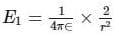Electric field at point P due to charge 8mc isin direction of 2mc and its value is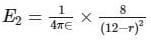for net field to be zero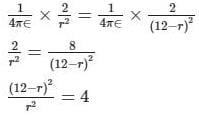taking root on both sides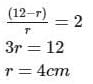Test: Electric Field Intensity & Electric Field Calculations - Question 2

### Two equal positive charges q1 = q2 = 2.0 μC. are located at x = 0, y = 0.3 and x = 0 and y = -0.3 m respectively. What are the magnitude and direction of the total electric force (expressed in Newton and degrees counter clockwise w.r.t  x - axis) that q1 and q2 exert on a third charge Q = 4.0 μC  at x =0.4 and y = 0 m

Detailed Solution for Test: Electric Field Intensity & Electric Field Calculations - Question 2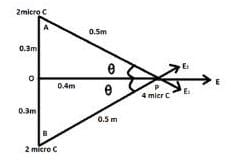Side AP =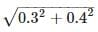= 0.5m Electric Field due to charge at A on point P = Elecric Field due to charge at B on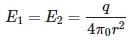point P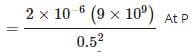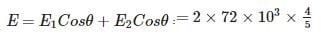Where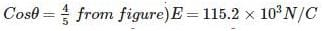So force due to this field on charge P

F = qE =  4 x 10-6 x 115.2 x 103 F = 0.46N

Since this force in the direction of positive x axis so angle  = 0o

Test: Electric Field Intensity & Electric Field Calculations - Question 3

### The electric field and force experienced by a test charge placed at origin is 6 x 105N/C and 18 x 10-4 N. The value of test charge is:​

Detailed Solution for Test: Electric Field Intensity & Electric Field Calculations - Question 3

By definition of Electric Field,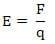Test: Electric Field Intensity & Electric Field Calculations - Question 4

A tennis ball which has been covered with charges is suspended by a thread so that it hangs between two metal plates. One plate is earthed, while other is attracted to a high voltage generator. The ball

Detailed Solution for Test: Electric Field Intensity & Electric Field Calculations - Question 4

The plate which connected to high voltage generator induces negative charge on ball which causes attraction. When the ball strikes the positive plate, charge distribution again takes place that is the bass becomes positive and repulsion takes place. When it strikes the plate which connected to earth than its charge goes to earth and again it will be attracted towards positive plate. Hence the ball swings backward and forward hitting each plate in turn.

Test: Electric Field Intensity & Electric Field Calculations - Question 5

Which, among the following is the correct expression for electric field?

Detailed Solution for Test: Electric Field Intensity & Electric Field Calculations - Question 5

Electric field is the force per unit charge hence, the correct expression is: E=F/Q.

Test: Electric Field Intensity & Electric Field Calculations - Question 6

A charge q is placed at the center of the line joining two equal charges Q. The system of the three charges will be in equilibrium if q is equal to

Detailed Solution for Test: Electric Field Intensity & Electric Field Calculations - Question 6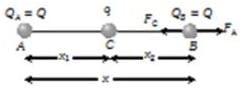Suppose in the following figure, equilibrium of charge B is considered.

Hence for its equilibrium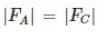Test: Electric Field Intensity & Electric Field Calculations - Question 7

Electric field intensity varies with distance as:

Detailed Solution for Test: Electric Field Intensity & Electric Field Calculations - Question 7

E = F/q = KQ/r2

Test: Electric Field Intensity & Electric Field Calculations - Question 8

If electric field lines cross each other that would mean

Detailed Solution for Test: Electric Field Intensity & Electric Field Calculations - Question 8

Properties of Electric Field Lines. At every point on an electric field line, the tangent represents the direction of electric field. If two field lines cross each other at the point, then there would be two possible directions for electric field which is physically impossible.

Test: Electric Field Intensity & Electric Field Calculations - Question 9

The S.I. unit of electric field intensity is:​

Detailed Solution for Test: Electric Field Intensity & Electric Field Calculations - Question 9

Electric Field Intensity E = F\q

Test: Electric Field Intensity & Electric Field Calculations - Question 10

The diagram shows the electric field lines in a region of space containing two small charged spheres, Y and Z then which statement is true?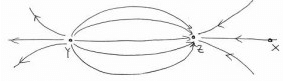Detailed Solution for Test: Electric Field Intensity & Electric Field Calculations - Question 10

A small negatively charged body placed at X would be pushed to the right

The negative charge feels a force opposite to the direction of field.

Other cases are not possible from properties of electric lines of force.

Test: Electric Field Intensity & Electric Field Calculations - Question 11

Which equation correctly shows the force on a charge q in an electric field E

Detailed Solution for Test: Electric Field Intensity & Electric Field Calculations - Question 11

E = F/q

Test: Electric Field Intensity & Electric Field Calculations - Question 12

A test charge of 3nC is placed at origin and experiences a force of 9 x 10-4 N. The electric field at origin is:​

Detailed Solution for Test: Electric Field Intensity & Electric Field Calculations - Question 12

E =F/q = (KQ/r2)

Test: Electric Field Intensity & Electric Field Calculations - Question 13

The field lines for single negative charge are:

Detailed Solution for Test: Electric Field Intensity & Electric Field Calculations - Question 13

Conventionally, field lines originate from a positive point charge and terminate at negative charge

Test: Electric Field Intensity & Electric Field Calculations - Question 14

At any point on S on an electric field line

Detailed Solution for Test: Electric Field Intensity & Electric Field Calculations - Question 14

When a tangent is drawn at any point on field line then that tangent gives the direction of electric field at that point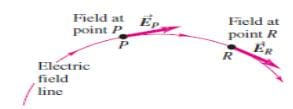Test: Electric Field Intensity & Electric Field Calculations - Question 15

An electric field can deflect

Detailed Solution for Test: Electric Field Intensity & Electric Field Calculations - Question 15

Only alpha rays are moving with small velocity and having charge so they will be affected by electric field.
X Rays and Gamma Rays are electromagnetic radiations. They do not carry electric charge while neutron is a charge less particle.

Test: Electric Field Intensity & Electric Field Calculations - Question 16

For uniform electric field, field lines are:

Detailed Solution for Test: Electric Field Intensity & Electric Field Calculations - Question 16

Uniform field lines imply that every point in space has same magnitude and direction of Electric Field. It is represented by parallel and equally spaced arrows in the direction of electric field

Test: Electric Field Intensity & Electric Field Calculations - Question 17

An electron traveling north enters a region where the electric field is uniform and points North.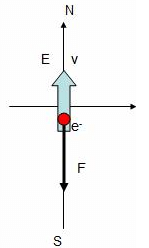Detailed Solution for Test: Electric Field Intensity & Electric Field Calculations - Question 17

Electron is negatively charged and force is always opposite to the direction of electric field. So the when the force acts along South and opposite to the velocity which means the electron slows down.

Test: Electric Field Intensity & Electric Field Calculations - Question 18

The magnitude of an electric field balancing a deutron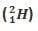of charge 1.6 x 10-19 C and mass 3.2 x 10-27 kg is:

Detailed Solution for Test: Electric Field Intensity & Electric Field Calculations - Question 18

Given:

Charge, q = 1.6 x 10-19 C

Mass, m = 3.2 x 10-27 kg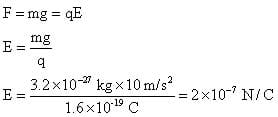Test: Electric Field Intensity & Electric Field Calculations - Question 19

Two charges A = -2.50 μCand B = 6.0 μC are at a distance of 1 meter from each other. Distance from A at which the electric field is zero in meters is

Detailed Solution for Test: Electric Field Intensity & Electric Field Calculations - Question 19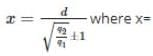distance of null point from small charge, d=distance between two charges, q2=bigger charge, q1=small charge. +1 for like charges and -1 for unlike charges x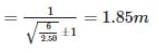Test: Electric Field Intensity & Electric Field Calculations - Question 20

Six charges are kept at the vertices of a regular hexagon as shown in the figure. If magnitude of forceapplied by +Q on +q charge is F, then net electric force on the +Q is: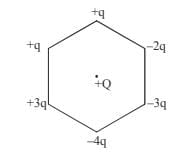Detailed Solution for Test: Electric Field Intensity & Electric Field Calculations - Question 20

Superposition of Electrostatic Force given by Coulomb’s Law for each of the charge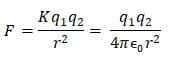## Physics Class 12

157 videos|452 docs|213 tests
 Use Code STAYHOME200 and get INR 200 additional OFF Use Coupon Code
Information about Test: Electric Field Intensity & Electric Field Calculations Page
In this test you can find the Exam questions for Test: Electric Field Intensity & Electric Field Calculations solved & explained in the simplest way possible. Besides giving Questions and answers for Test: Electric Field Intensity & Electric Field Calculations, EduRev gives you an ample number of Online tests for practice

## Physics Class 12

157 videos|452 docs|213 tests

### How to Prepare for NEET

Read our guide to prepare for NEET which is created by Toppers & the best Teachers